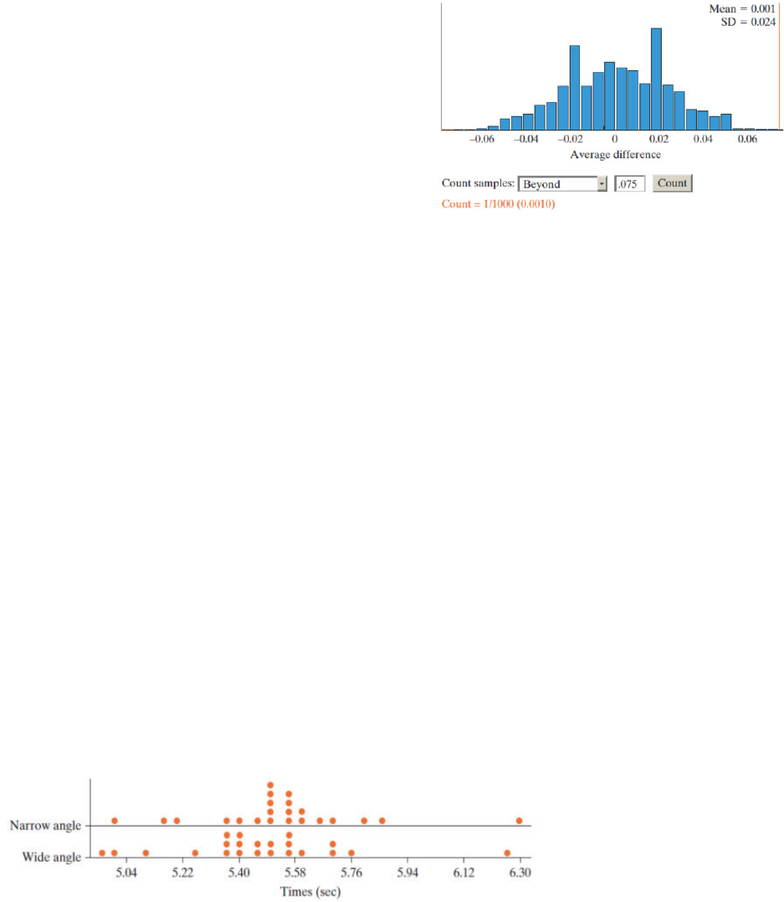Class Notes (1,100,000)
US (480,000)
UCLA (10,000)
STATS (200)
Lecture 22

# STATS 13 Lecture Notes - Lecture 22: Null Hypothesis, Random Assignment, Dependent And Independent Variables

Department
Statistics
Course Code
STATS 13
Professor
Tsiang, Mike
Lecture
22

This preview shows page 1. to view the full 4 pages of the document.Rounding First Base: Simulate!
null distribution of 1000 simulated mean dierences is
shown below!
where is the center!
where is our observed statistic of .075!
!
Rounding First Base: Strength of Evidence!
1 of the 1000 repetitions of random swapping gave a x
̄d
value at least as extreme as .075!
the simulation based p-value is .001!
we can also standardized .075 by dividing by the applet's
estimate of SD (x
̄d) approximately = to .024!
the observed statistic is t= .075-0 / .024 = 3.125!
!
Rounding First Base: Conclusions!
with a p value of .001 we have very strong evidence against the null hypothesis and can conclude that the
running path does matter with the wide angle path being faster on average !
we can draw a cause and eect conclusion since the reseacher used random assignment of he two base running
methods for each runner!
there was no much information about how the 22 runners were selected to decide if we can generalize to a larger
population !
!
The 3S Strategy!
statistic: compute the statistic in the sample. in this case the statistic we looked at was the observed mean
dierence in running times!
simulate: identify a chance model that reﬂects the null hypothesis. we tossed a coin for each runner and if it
landed heads we swapped the two running times for the under. if the coin landed tails we did not swap the times.
compute the mean dierence for the 22 runners and expected this process many times!
strength of evidence: 1 out of 1000 of our simulated mean dierences was at least as extreme as the observed
dierence of .075 seconds!
!
Rounding First Base: Estimation!
an approximation 95% conﬁdence interval for μd:!
.075 ± 2 X .024 = .075 ± .048 seconds!
or (.027, .124)!
interpretation: we are 95% conﬁdent that, on average, the narrow angle route takes .027 to .124 seconds longer
than the wide angle route!
!
Rounding First Base: Alternative Analysis!
what if we incorrectly analyzed the data using a two independent samples procedure!
suppose the researcher selected 22 runners to use the wide method and an independent sample of 22 other
runners to use the narrow method ,obtaining the same 44 running times as in the !
!
!
!
!
!
!
!
!
Rounding First Base: Alternative Analysis!
using applet, we get a p-value of .3470!
does it make sense that this p value is larger than the one we obtained earlier!
!
Section 7.3: Theory Based Approach for Analyzing Paired Data!
!
Example 7.3 How many M&Ms Would you Like?!
does bowl size aect how much you eat!
17 participants were assigned to receive either a small or large bowl and were allowed to take as many M&Ms as